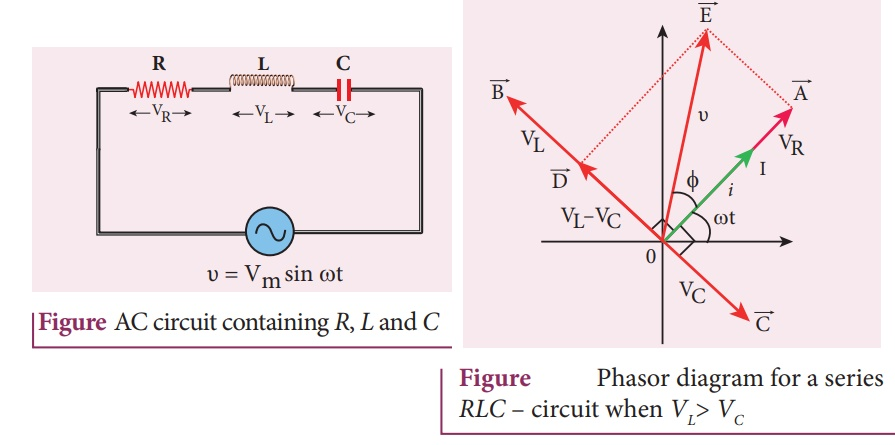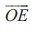Home | | Physics 12th Std | AC circuit containing a resistor, an inductor and a capacitor in series - Series RLC circuit

# AC circuit containing a resistor, an inductor and a capacitor in series - Series RLC circuitConsider a circuit containing a resistor of resistance R, a inductor of inductance L and a capacitor of capacitance C connected across an alternating voltage source.

AC circuit containing a resistor, an inductor and a capacitor in series - Series RLC circuit

Consider a circuit containing a resistor of resistance R, a inductor of inductance L and a capacitor of capacitance C connected across an alternating voltage source (Figure 4.51). The applied alternating voltage is given by the equation.Let i be the resulting circuit current in the circuit at that instant. As a result, the voltage is developed across R, L and C. We know that voltage across R (VR) is in phase with i, voltage across L (VL) leads i by π/2 and voltage across C (VC) lags i by π/2.

The phasor diagram is drawn with current as the reference phasor. The current is represented by the phasoras shown in Figure 4.52.The length of these phasors are

OI = Im, OA = ImR, OB = ImXL; OC = ImXC

The circuit is either effectively inductive or capacitive or resistive that depends on the value of VL or VC. Let us assume that VL > VC so that net voltage drop across L-C combination is VLVC which is represented by a phasor.

By parallelogram law, the diagonalgives the resultant voltage υ of VR and (VLVC) and its length OE is equal to Vm. Therefore,Z is called impedance of the circuit which refers to the effective opposition to the circuit current by the series RLC circuit. The voltage triangle and impedance triangle are given in the Figure 4.53.From phasor diagram, the phase angle between υ and i is found out from the following relationSpecial cases

(i) If XL > XC, (XLXC) is positive and phase angle ϕ is also positive. It means that the applied voltage leads the current by ϕ (or current lags behind voltage by ϕ). The circuit is inductive.

υ = Vm sin ωt; i = I m sin( ω t −φ)

(ii) If XL < XC, (XLXC) is negative and ϕ is also negative. Therefore current leads voltage by ϕ and the circuit is capacitive.

υ = Vm sin ωt;  i = I m sin( ω t + φ)

(iii) If XL = XC, ϕ is zero. Therefore current and voltage are in the same phase and the circuit is resistive.υ =Vm sin ωt;   i = Im sin ωt

Tags : Phasor diagram, Circuit Diagram, Formula, Solved Example Problems | Alternating Current (AC) , 12th Physics : Electromagnetic Induction and Alternating Current
Study Material, Lecturing Notes, Assignment, Reference, Wiki description explanation, brief detail
12th Physics : Electromagnetic Induction and Alternating Current : AC circuit containing a resistor, an inductor and a capacitor in series - Series RLC circuit | Phasor diagram, Circuit Diagram, Formula, Solved Example Problems | Alternating Current (AC)

Related Topics